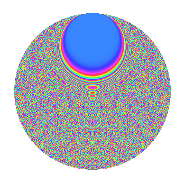# Properties

 Label 3234.2.ccLevel 3234 Weight 2 Character orbit cc Rep. character $$\chi_{3234}(125,\cdot)$$ Character field $$\Q(\zeta_{70})$$ Dimension 5376 Sturm bound 1344

# Related objects

## Defining parameters

 Level: $$N$$ $$=$$ $$3234 = 2 \cdot 3 \cdot 7^{2} \cdot 11$$ Weight: $$k$$ $$=$$ $$2$$ Character orbit: $$[\chi]$$ $$=$$ 3234.cc (of order $$70$$ and degree $$24$$) Character conductor: $$\operatorname{cond}(\chi)$$ $$=$$ $$1617$$ Character field: $$\Q(\zeta_{70})$$ Sturm bound: $$1344$$

## Dimensions

The following table gives the dimensions of various subspaces of $$M_{2}(3234, [\chi])$$.

Total New Old
Modular forms 16320 5376 10944
Cusp forms 15936 5376 10560
Eisenstein series 384 0 384

## Trace form

 $$5376q - 224q^{4} + 4q^{7} + O(q^{10})$$ $$5376q - 224q^{4} + 4q^{7} + 224q^{16} + 16q^{18} + 20q^{21} + 10q^{22} + 216q^{25} + 126q^{27} + 6q^{28} + 8q^{37} - 40q^{39} - 42q^{40} + 108q^{42} + 64q^{43} - 28q^{45} + 152q^{49} + 24q^{51} + 14q^{55} - 88q^{57} + 230q^{58} + 42q^{63} - 224q^{64} + 64q^{67} - 56q^{69} - 16q^{70} + 24q^{72} + 32q^{78} - 88q^{79} + 36q^{81} + 96q^{85} + 112q^{87} + 4q^{88} - 184q^{91} + 52q^{93} + 336q^{94} - 736q^{99} + O(q^{100})$$

## Decomposition of $$S_{2}^{\mathrm{new}}(3234, [\chi])$$ into newform subspaces

The newforms in this space have not yet been added to the LMFDB.

## Decomposition of $$S_{2}^{\mathrm{old}}(3234, [\chi])$$ into lower level spaces

$$S_{2}^{\mathrm{old}}(3234, [\chi]) \cong$$ $$S_{2}^{\mathrm{new}}(1617, [\chi])$$$$^{\oplus 2}$$

## Hecke characteristic polynomials

There are no characteristic polynomials of Hecke operators in the database